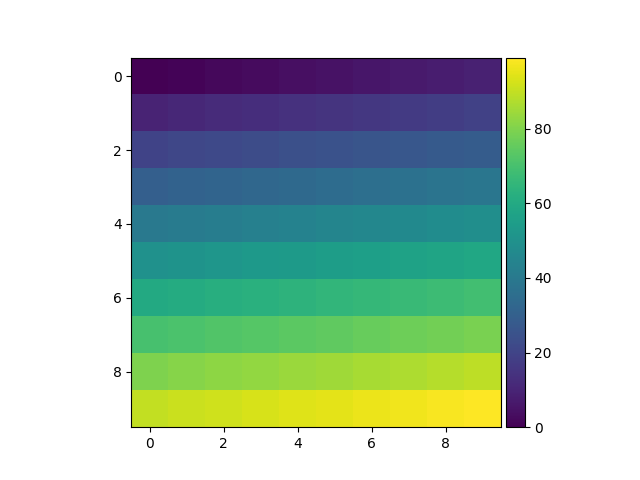Simple Colorbar¶Out:

<matplotlib.colorbar.Colorbar object at 0x7f188ba40c70>

import matplotlib.pyplot as plt
from mpl_toolkits.axes_grid1 import make_axes_locatable
import numpy as np

ax = plt.subplot(111)
im = ax.imshow(np.arange(100).reshape((10, 10)))

# create an axes on the right side of ax. The width of cax will be 5%
# of ax and the padding between cax and ax will be fixed at 0.05 inch.
divider = make_axes_locatable(ax)